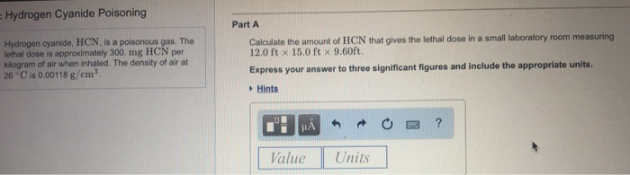# Problem: Hydrogen cyanide, HCN, is a poisonous gas. The ethal dose is approximately 300 mg HCN per kilogram of air when inhaled. The density of air at 26°C is 0.00118 g/cm3. Calculate the amount of HCN that gives the lethal dose in a small laboratory room measuring 12.0 ft x 15.0 ft × 960 ft. Express your answer to three significant figures and include the appropriate units.

###### FREE Expert Solution
97% (263 ratings)###### Problem Details

Hydrogen cyanide, HCN, is a poisonous gas. The ethal dose is approximately 300 mg HCN per kilogram of air when inhaled. The density of air at 26°C is 0.00118 g/cm3

Calculate the amount of HCN that gives the lethal dose in a small laboratory room measuring 12.0 ft x 15.0 ft × 960 ft.

Express your answer to three significant figures and include the appropriate units.